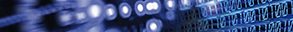Journal metrics
Acceptance rate13%
Submission to final decision81 days
Acceptance to publication56 days
CiteScore0.510
Impact Factor-

### Multiparameter-Based Fuzzy Logic Health Index Assessment for Oil-Immersed Power Transformers

####Journal profile

Advances in Fuzzy Systems provides an international forum for original research articles in the theory and applications of fuzzy subsets and systems.

####Editor spotlight

Advances in Fuzzy Systems maintains an Editorial Board of practicing researchers from around the world, to ensure manuscripts are handled by editors who are experts in the field of study.

####Special Issues

Do you think there is an emerging area of research that really needs to be highlighted? Or an existing research area that has been overlooked or would benefit from deeper investigation? Raise the profile of a research area by leading a Special Issue.

## Latest Articles

More articlesResearch Article

### The Measure of Fuzzy Filters on BL-Algebras

The new concept of the fuzzy filter degree was given by means of the implication operator, which enables to measure a degree to which a fuzzy subset of a BL-algebra is a fuzzy filter. In this paper, we put forward several equivalent characterizations of the fuzzy filter degree by studying its properties and the relationship with level cut sets. Furthermore, we study the fuzzy filter degrees of the intersection and fuzzy direct products of fuzzy subsets and investigate the fuzzy filter degrees of the image and the preimage of a fuzzy subset under a homomorphism.

Research Article

### Fuzzy Fractional Evolution Equations and Fuzzy Solution Operators

In this paper, the fuzzy fractional evolution equations of order q (FFEE) with fuzzy Caputo fractional derivative are introduced. We study the existence and uniqueness of mild solutions for FFEE under some conditions. Also, we generalize the definition of the fuzzy fractional integral and derivative order q. The fuzzy Laplace transform is presented and proved. The solvability of the problem (FFEE) and the properties of the fuzzy solution operator and its generator are investigated and developed.

Research Article

### Fuzzy Ideals and Fuzzy Filters of Pseudocomplemented Semilattices

In this paper, we introduce the concept of kernel fuzzy ideals and ⁎-fuzzy filters of a pseudocomplemented semilattice and investigate some of their properties. We observe that every fuzzy ideal cannot be a kernel of a ⁎-fuzzy congruence and we give necessary and sufficient conditions for a fuzzy ideal to be a kernel of a ⁎-fuzzy congruence. On the other hand, we show that every fuzzy filter is the cokernel of a ⁎-fuzzy congruence. Finally, we prove that the class of ⁎-fuzzy filters forms a complete lattice that is isomorphic to the lattice of kernel fuzzy ideals.

Research Article

### Predictive Control for Interval Type-2 Fuzzy System with Event-Triggered Scheme

In this paper, a synthesis approach of model predictive control (MPC) is proposed for interval type-2 (IT2) Takagi-Sugeno (T-S) fuzzy system with quantization error, bounded disturbance, and data loss. The novelty lies in the following technical improvements. In order to reduce the redundant data transmission, an event-triggered communication scheme is applied to determine whether the control law should be transmitted into the communication network or not. The IT2 T-S fuzzy model is utilized to address the nonlinearity of plant with parameter uncertainties, which can be captured by the lower and upper membership functions. Furthermore, the phenomena of data loss and quantization error between the controller and the actuator are expressed as Markovian chain and sector-bound uncertainties. The synthesis approach of MPC is provided by solving an MPC optimization problem over an infinite horizon objective function which explicitly considers the input constraints. By applying the quadratic boundedness (QB) technique, the recursive feasibility and quadratic stability of closed-loop system can be guaranteed. A numerical simulation and comparison studies are proposed to illustrate the effectiveness of this approach.

Research Article

### Existence and Continuous Dependence on Initial Data of Solution for Initial Value Problem of Fuzzy Multiterm Fractional Differential Equation

In this paper, the fuzzy multiterm fractional differential equation involving Caputo-type fuzzy fractional derivative of order is considered. The uniqueness of solution is established by using the contraction mapping principle and the existence of solution is obtained by Schauder fixed point theorem.

Research Article

### An Estimation of a Hybrid Log-Poisson Regression Using a Quadratic Optimization Program for Optimal Loss Reserving in Insurance

In this article, we are interested in developing an alternative estimation method of the parameters of the hybrid log-Poisson regression model. In our previous paper, we have proposed a hybrid log-Poisson regression model where we have derived the analytical expression of the fuzzy parameters. We found that the hybrid model provide better results than the classical log-Poisson regression model according to the mean square error prediction and the goodness of fit index. However, nowhere we have taken into account the optimal value of (cut) which is of greatest importance in fuzzy regressions literature. In this paper, we provide an alternative estimation method of our hybrid model using a quadratic optimization program and the optimized value (cut). The expected value of fuzzy number is used as a defuzzification procedure to move from fuzzy values to crisp values. We perform the hybrid model with the alternative estimation we are suggesting on two different numerical data to predict incremental payments in loss reserving. From the mean square error prediction, we prove that the alternative estimation of the new hybrid model with an optimized value predicts incremental payments better than the classical log-Poisson regression model as well as the same hybrid model with analytical estimation of parameters. Hence we have optimized the outstanding loss reserves.Journal metrics
Acceptance rate13%
Submission to final decision81 days
Acceptance to publication56 days
CiteScore0.510
Impact Factor-